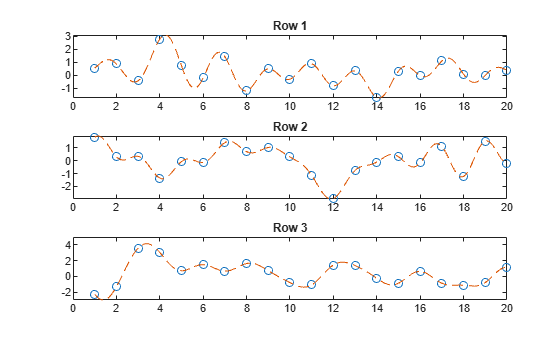# interpft

1-D interpolation (FFT method)

## Syntax

``y = interpft(X,n)``
``y = interpft(X,n,dim)``

## Description

example

````y = interpft(X,n)` interpolates the Fourier transform of the function values in `X` to produce `n` equally spaced points. `interpft` operates on the first dimension whose size does not equal 1.```

example

````y = interpft(X,n,dim)` operates along dimension `dim`. For example, if `X` is a matrix, then `interpft(X,n,2)` operates on the rows of `X`.```

## Examples

collapse all

Interpolate 1-D data using the FFT method and visualize the result.

Generate some sample points in the interval $\left[0,3\pi \right]$ for the function $f\left(x\right)={\mathrm{sin}}^{2}\left(x\right)\mathrm{cos}\left(x\right)$. Use a spacing interval `dx` to ensure the data is evenly spaced. Plot the sample points.

```dx = 3*pi/30; x = 0:dx:3*pi; f = sin(x).^2 .* cos(x); plot(x,f,'o')```Use FFT interpolation to find the function value at 200 query points.

```N = 200; y = interpft(f,N);```

Calculate the spacing of the interpolated data from the spacing of the sample points with `dy = dx*length(x)/N`, where `N` is the number of interpolation points. Truncate the data in `y` to match the sampling density of `x2`.

```dy = dx*length(x)/N; x2 = 0:dy:3*pi; y = y(1:length(x2));```

Plot the results.

```hold on plot(x2,y,'.') title('FFT Interpolation of Periodic Function')```Generate three separate data sets of normally distributed random numbers. Assume the data is sampled at the positive integers, `1:N`. Store the data sets as rows in a matrix.

```A = randn(3,20); x = 1:20;```

Interpolate the rows of the matrix at 500 query points each. Specify `dim = 2` so that `interpft` works on the rows of `A`.

```N = 500; y = interpft(A,N,2);```

Calculate the spacing interval of the interpolated data `dy`. Truncate the data in `y` to match the sampling density of `x2`.

```dy = length(x)/N; x2 = 1:dy:20; y = y(:,1:length(x2));```

Plot the results.

```subplot(3,1,1) plot(x,A(1,:)','o'); hold on plot(x2,y(1,:)','--') title('Row 1') subplot(3,1,2) plot(x,A(2,:)','o'); hold on plot(x2,y(2,:)','--') title('Row 2') subplot(3,1,3) plot(x,A(3,:)','o'); hold on plot(x2,y(3,:)','--') title('Row 3')```## Input Arguments

collapse all

Input array, specified as a vector, matrix, or multidimensional array. The data in `X` is assumed to be sampled at an evenly spaced interval of the independent variable. `interpft` works best with periodic data.

Data Types: `single` | `double`
Complex Number Support: Yes

Number of points, specified as a positive integer scalar.

Data Types: `single` | `double`

Dimension to operate along, specified as a positive integer scalar. If no value is specified, then the default is the first array dimension whose size does not equal 1.

• `interpft(X,n,1)` interpolates the columns of `X`.• `interpft(X,n,2)` interpolates the rows of `X`.Example: `interpft(X,n,3)`

## Output Arguments

collapse all

Interpolated points, returned as a vector, matrix, or multidimensional array. If `length(X,dim) = m`, and `X` has a sampling interval of `dx`, then the new sampling interval for `y` is `dy = dx*m/n`, where `n > m`.

If `dim` is specified, then `interpft` pads or truncates `X` to length `n` in dimension `dim`, so that `size(y,dim) = n`.

## Algorithms

The `interpft` function uses the FFT method. The original vector `x` is transformed to the Fourier domain using `fft`, and then it is transformed back with more points.

## Version History

Introduced before R2006a